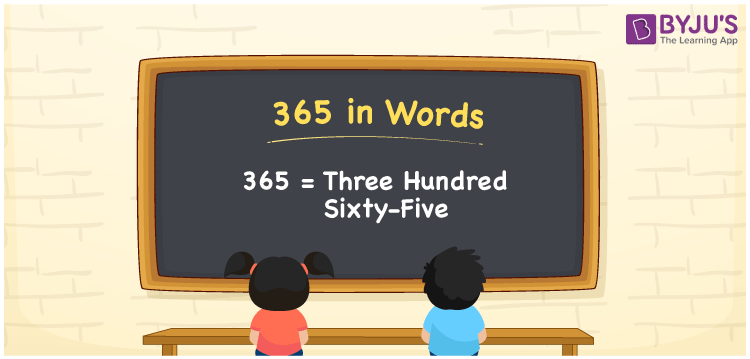# 365 in Words

We can write the number 365 in words as Three Hundred Sixty-Five. For example, Jasmine purchased a book worth Rs.365, then you can write, “Jasmine purchased a book worth Rupees Three Hundred Sixty-Five”. Generally, in Mathematics, we write the number names with the help of a place value chart. The number 365 is a cardinal number since it shows a certain amount.

 365 in Words Three Hundred Sixty-Five Three Hundred Sixty-Five in numerical form 365

## 365 in English Words

Generally, the English alphabet is used to represent numbers in words. Hence, we can read and write the number 365 in English as Three Hundred Sixty-Five.## How to Write 365 in Words?

First, we have to check the position of each digit of the given number before converting it into words. 365 is a three-digit number. Hence, let us create a chart of 3 columns as mentioned below.

 Hundreds Tens Ones 3 6 5

Therefore, we can write the expanded form as:

3 x Hundred + 6 x Ten + 5 x One

= 3 x 100 + 6 x 10 + 5 x 1

= 300 + 60 + 5

= 365

= Three Hundred Sixty-Five

Hence, 365 in words is written as Three Hundred Sixty-Five

Interesting way of writing 365 in words

3 = Three

36 = Thirty-Six

365 = Three Hundred Sixty-Five

Thus, the word form of the number 365 is Three Hundred Sixty-Five

365 is a natural number that is one less than 366 and one greater than 364

• 365 in words – Three Hundred Sixty-Five
• Is 365 an odd number? – Yes
• Is 365 an even number? – No
• Is 365 a perfect square number? – No
• Is 365 a perfect cube number? – No
• Is 365 a prime number? – No
• Is 365 a composite number? – Yes

## Frequently Asked Questions on 365 in Words

Q1

### Write 365 in English words.

We can write 365 in English words as Three Hundred Sixty-Five.
Q2

### Simplify 300 + 65, and express in words.

Simplifying 300 + 65, we get 365. Hence, 365 in words is Three Hundred Sixty-Five.
Q3

### What is the place value of 3 in 365?

The place value of 3 in 365 is Hundred.